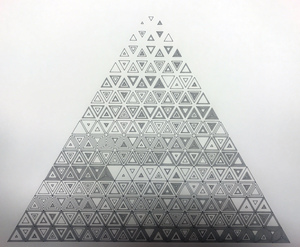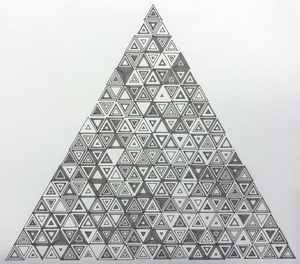If you place equilateral triangles in a triangular pattern you get one in row one, three in row two, five in row three, seven in row four and so on. In every row an odd number of triangles is added. It is not very hard to prove that if you add the first n odd numbers you get n(Σ2n-1=n2). With an 8 bits number you get 28 = 256 numbers. The square root of 256 is of course 24 = 16 so you can make a triangle with 16 rows! Well That’s the mathematical story. Maybe you just like them.

#####8 bits triangles, black on white, in sequence (nr. 09-01)
• 8 bits so 28 = 256 triangles
• Plotted on CANSON® XL® Bristol Bristolpaper A4.
• Black pigmented fineliner
• In sequence from the top to the bottom right
• Dimensions: 22x19cm

#####8 bits triangles, black on white, shuffled (nr. 09-02)
• 8 bits so 28 = 256 triangles
• Plotted on CANSON® XL® Bristol Bristolpaper A4.
• Black pigmented fineliner
• Shuffled, all triangles are different
• Dimensions: 22x19cm

#####6 bits triangles, black on white, shuffled
• 6 bits so 26 = 64 triangles
• Plotted on CANSON® XL® Bristol Bristolpaper A5.
• Black pigmented fineliner
• Shuffled, all triangles are different
• Dimensions: 15x13cm